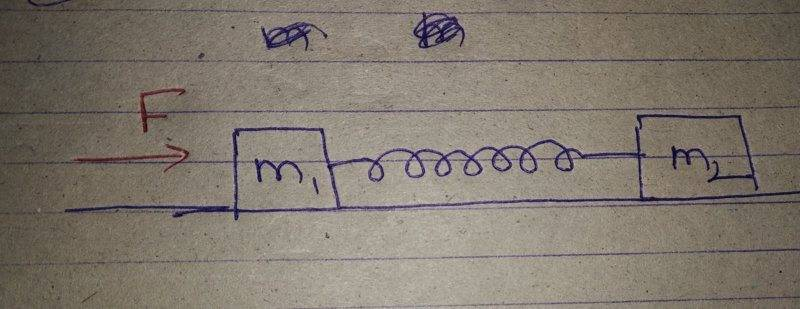# Will the block m2 move?

• B
Gold MemberSuppose two blocks m1 and m2,connected by a spring are kept on a frictionless surface and the block m1 is applied an external force F.
My teacher taught me that in a spring the force is generated in the direction opposite to the displacement of the spring.
So the spring would generate a force in the left direction as it's displacement would be right.
So my question is that will the block m2 move in the *left* direction or not as a force is getting applied on it in the left direction?
I will be thankful for help!

#### Attachments

stockzahn
Homework Helper
Gold Member
What would happen, if the mass of block ##m_2## would be zero? What would be the force on ##m_1##? Try to apply Newton's 3rd law.

Gold Member
If mass of m2 is zero then block m1 would just move with acceleration F/m1.
What are you trying to explain?
What would happen, if the mass of block ##m_2## would be zero? What would be the force on ##m_1##? Try to apply Newton's 3rd law.

stockzahn
Homework Helper
Gold Member
If mass of m2 is zero then block m1 would just move with acceleration F/m1.
What are you trying to explain?

There only would be a force to the left acting on ##m_1## if there is a "counterforce" acting to the right on ##m_2##. From the perspective of ##m_2## the displacement of the spring would be to the left.

A.T.
My teacher taught me that in a spring the force is generated in the direction opposite to the displacement of the spring.
Have you never compressed/stretched a spring with your hands? It should be obvious that it applies two opposite forces at both ends.

sophiecentaur